# How to calculate 95% confidence interval in Excel

You can watch a video tutorial here.The 95% confidence interval is used in statistics to define a range of values that you can be 95%  confident of containing the mean of the population. Excel has a Data Analysis add-in that can help you calculate the 95% confidence interval.

Note: If the Data Analysis button is present under Data > Analyze,  then skip steps 1 to 3

### Step 1 – Open the Excel options window– Go to File > Options

### Step 2 – Manage the Add-ins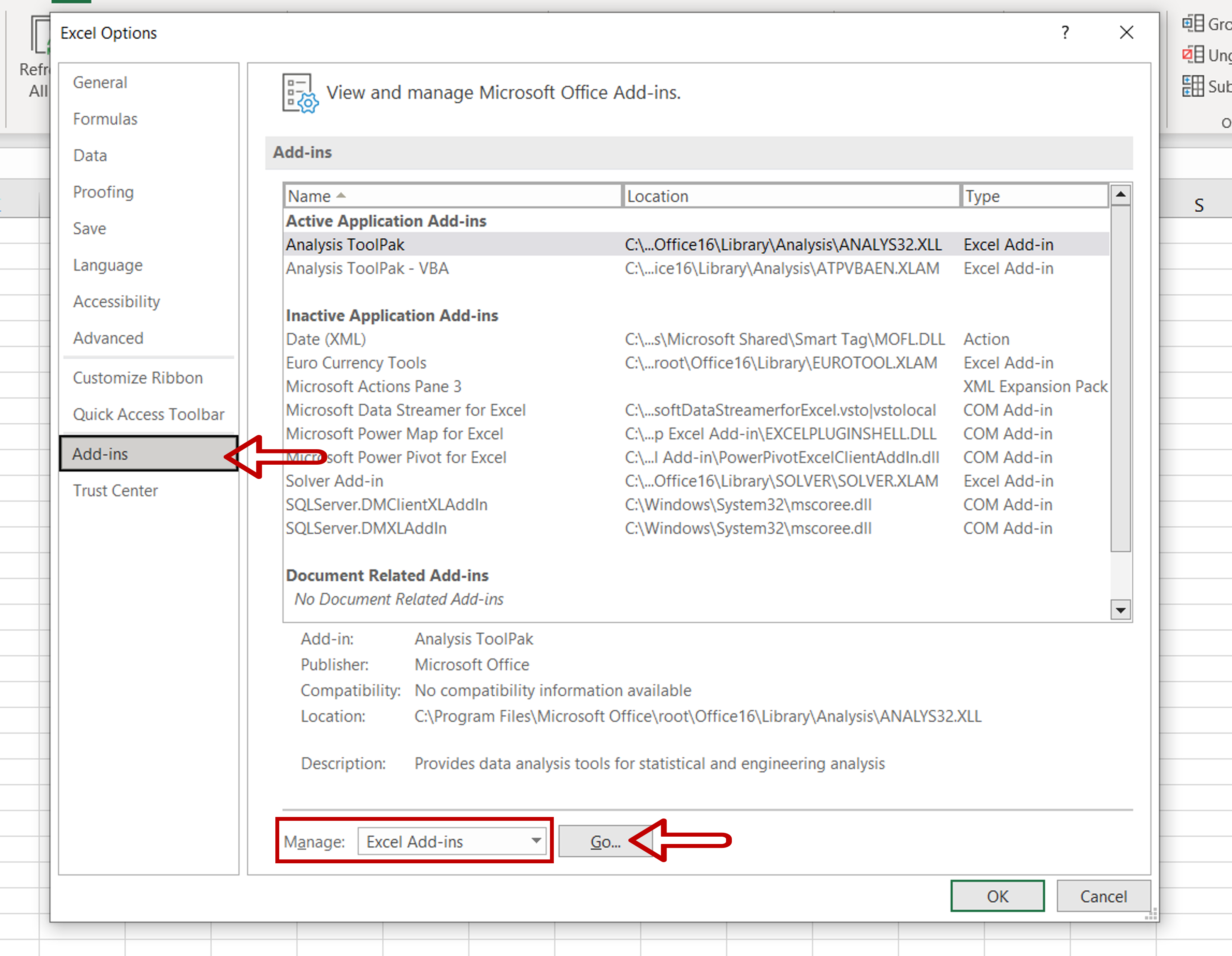– Select Excel Add-ins from the Manage drop-down
– Click Go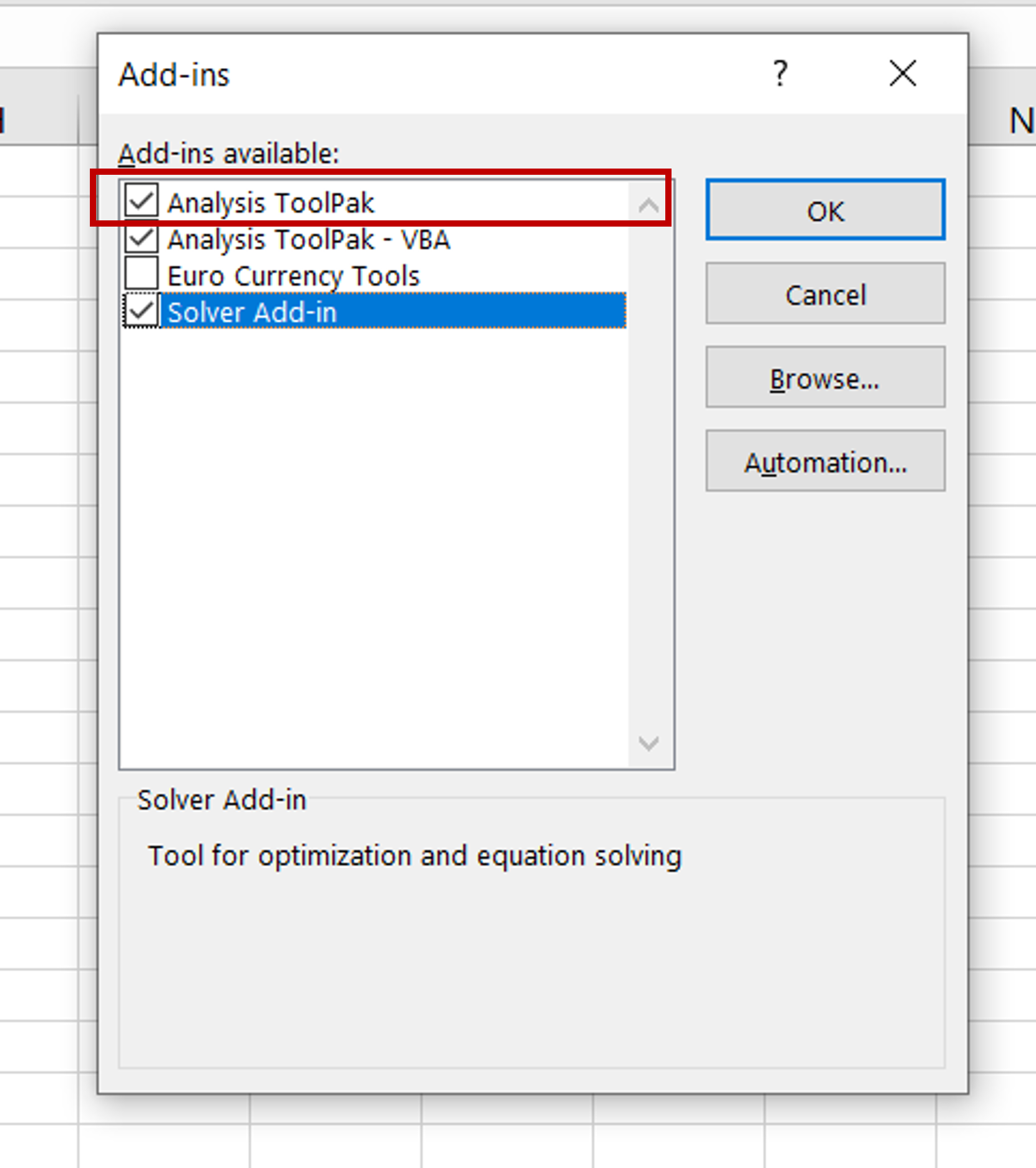– Select Analysis ToolPak
– Click OK

### Step 4 – Open the Data Analysis window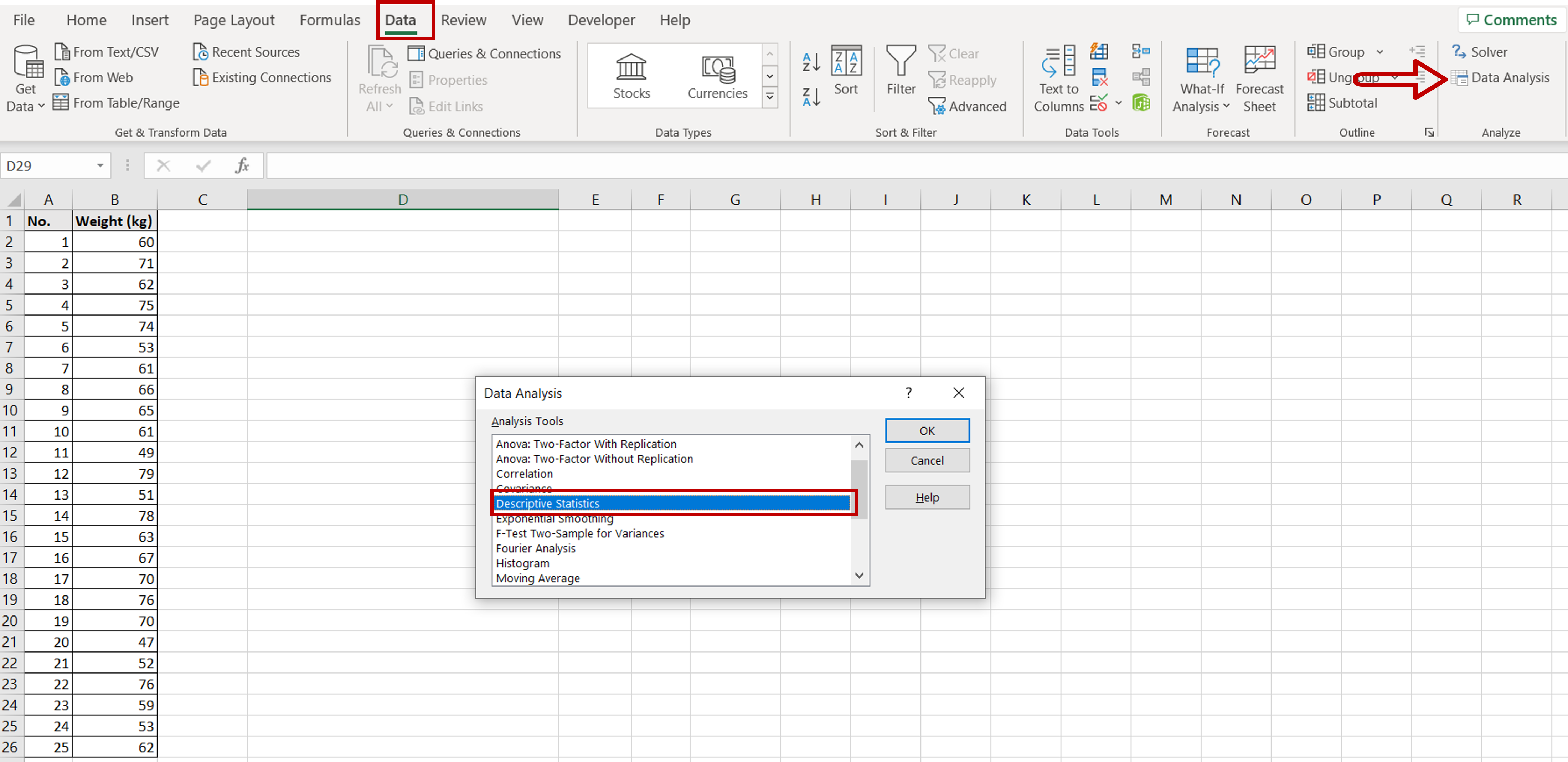– Go to Data > Analyze
– Click on the Data Analysis button
– In the window, select Descriptive Statistics
– Click OK

### Step 5 – Set the parameters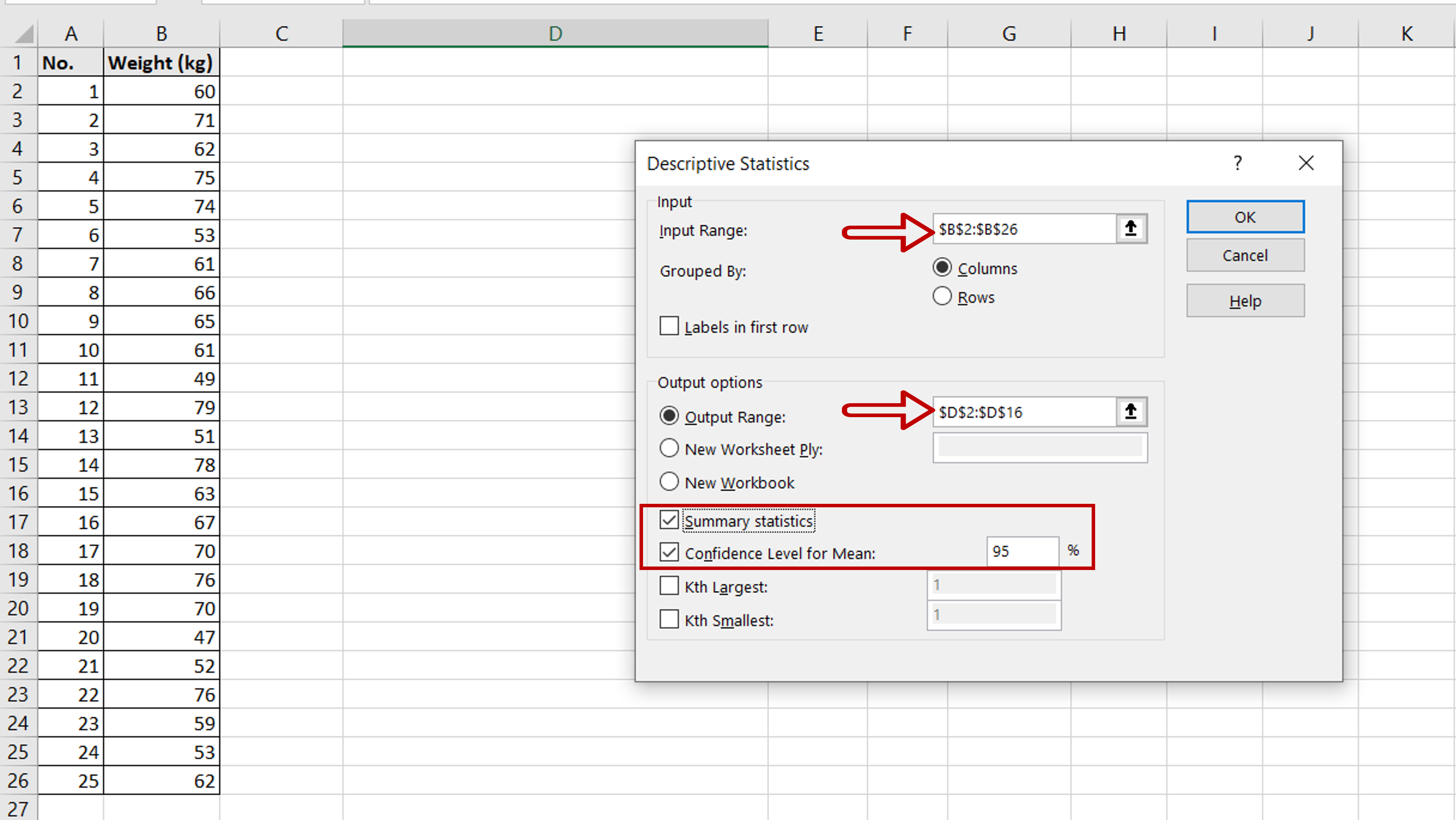– Define the input and output ranges
– Tick the Summary statistics check-box
– Tick the Confidence Level for Mean check-box and set the percentage as 95
– Click OK

### Step 6 – Compute the 95% confidence interval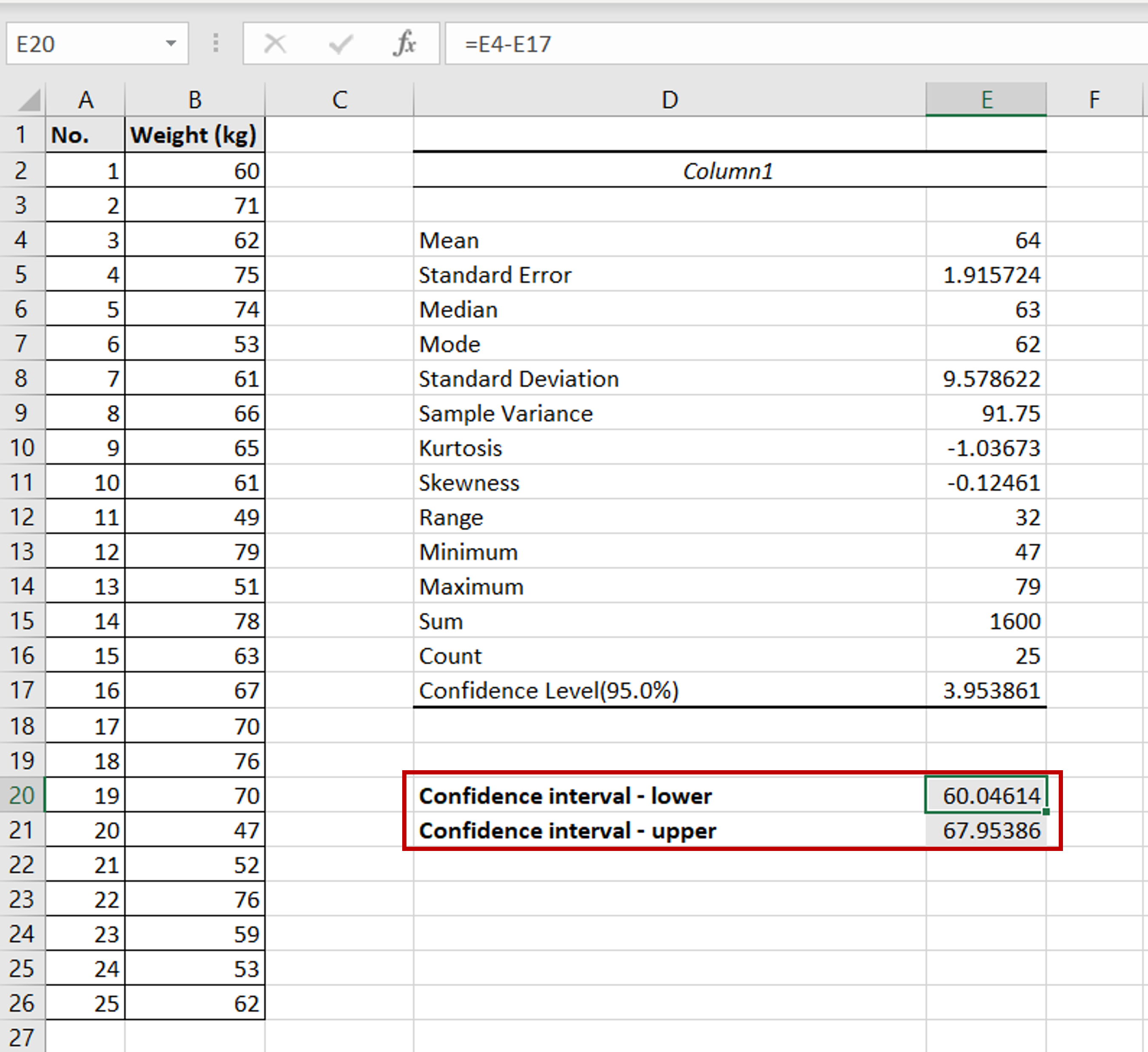– Enter the formula for the lower limit of the confidence interval:
=<cell reference of the ‘Mean’> – <cell reference of the ‘Confidence Level(95.0%)’>
– Enter the formula for the upper limit of the confidence interval:
=<cell reference of the ‘Mean’> + <cell reference of the ‘Confidence Level(95.0%)’>